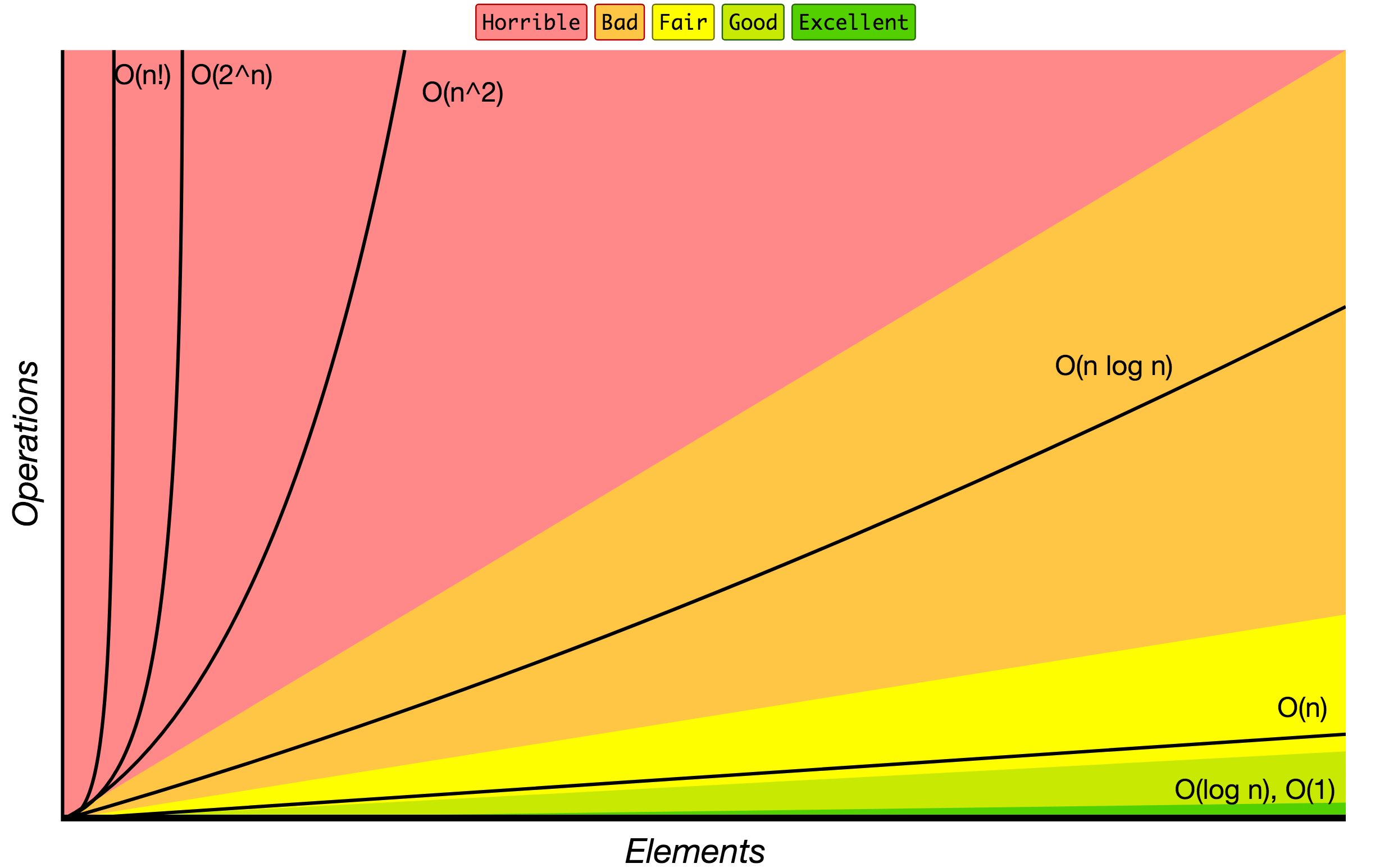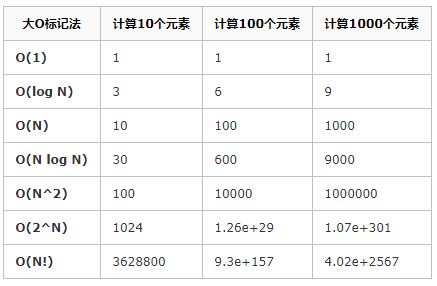• 本篇文章重点分析如何计算一个程序时间复杂度和空间复杂度 一、时间复杂度 对涉及的对数时间复杂度写法的说明： 平时我们计算时间复杂度常说的logn以及nlog都没有涉及底数，这是因为无论是以e为底，还是以某个数...
理解程序执行的时间复杂度和空间复杂度对于优化程序非常重要 本篇文章重点分析如何计算一个程序的时间复杂度和空间复杂度 一、时间复杂度 对涉及的对数时间复杂度写法的说明： 平时我们计算时间复杂度常说的logn以及nlog都没有涉及底数，这是因为无论是以e为底，还是以某个常数为底，底数始终是一个常数，在计算时间复杂度时常数可以忽略，因此，习惯性的写为logn、nlogn等等。 概念 执行算法所需要消耗的时间长短，称为算法的时间复杂度。 时间复杂度的表示方式(大O) T(n)=O(f(n)) n是影响复杂度变化的因子，f(n)是复杂度具体的算法。 常见的时间复杂度量级(从小到大) 常数阶:O(1)； 对数阶:O(logn) 线性阶:O(n)； 线性对数阶:O(nlogn) 平方阶:O(n2) 立方阶:O(n3) k次方阶:O(nk) 指数阶:O(2n) 阶乘阶:O(n!) 下面为了方便理解这些阶数的大小，给出博客(https://www.cnblogs.com/chenjinxinlove/p/10038919.html) 中绘制的时间复杂度曲线以及性能比较。举例分析 常数阶
int x=1;
int y=7;
y=x;

时间复杂度表示的是代码执行时间的一个增长变化趋势，在上面的代码中无论出现多少个赋值语句，其时间复杂度仍为O(1)。这是由于赋值操作本身没有增长趋势。 对数阶
int i=1;
while(i<n){
i*=2;
}

对于上面的代码，假设n=16，则循环实际上一共执行4次，24=16，log216=4。所以时间复杂度为O(logn)，至于为什么不写成O(lon2n)，在文章的开头已经说明，这里不再赘述。 线性阶
int i=0;
int j=0;
while(i<n){
i++;
j++;
}

利用上面的代码顺便解决一个疑惑， 为什么时间复杂度都是统一的形式，不需要加常数项或者倍数 对于上述第1、2行代码，各执行一次；第三行代码执行1次；第4、5行代码各执行n次，加起来整个程序一共执行了2n+3次，那时间复杂度是不是直接写O(2n+3)就好了？？ 开始我也这么觉得，但是本文章上面提到，时间复杂度表示的是时间增长的一个趋势，占据算法运行时间长短的是它的增长趋势，1表示常数阶，n表示线性阶等等，因此没有~~O(2n+3)~~这种写法，只有O(n)，统一写为O(n)也可以更好地区分，只要时间复杂度都为O(n)，除去最好和最坏的时间，其算法的运行时间在一个数量级上，相差不会太大。 线性对数阶
int i=0;
int j=1;
while(i<n){
while(j<n){
j*=2;
}
i++;
}

上面算法有两层循环，最外层执行n次，里面一层执行logn次，因此算法事件复杂度为O(nlogn)。这两个算法的代码在上面都分析过，只不过在此进行嵌套。 平方阶
int i=0;
int j=0;
while(i<n){
while(j<n){
j++;
}
i++;
}

对于上面已经提到的线性阶O(n)，两个线性阶进行嵌套，得到的就是平方阶，当然两层循环不一定都用n表示，改成m也是平方阶。趋势！趋势！趋势！很重要。 立方阶、k次方阶，在这里不再举例，就是对线性阶的多层嵌套 对于后面的指数阶和阶乘阶在这里不好给出例子(我没找到合适的简单概括这两个例子的代码)，恳请各位大神在评论区补充，我看到后一定立马补充进来，谢谢你们。 二、空间复杂度 概念 算法执行所需要消耗的存储空间的大小，称为算法的空间复杂度 空间复杂度主要包含三个方面： 1.程序本身所占空间 2.输入数据所占空间； 3.辅助变量所占空间 这个空间复杂度的理解可以对比时间复杂度来理解和计算 空间复杂度的表示方式(大O) S(n)=O(g(n)) n表示问题规模 举例分析 常数阶
int i=0;
int j=0;
int sum=0;
i+=100;
j-=1000;
sum=i+j;

对上面的代码分析可知，代码中所需要的临时空间不会随着某个变量的改变而增加存储空间，因此算法的空间复杂度是一个常数阶O(1)。 线性阶
vector<int> vec=(n,1);
for(int i=0;i<n;i++)vec.push_back(i);

对于上面的代码，第1行是定义并初始化一个一维向量，向量大小所占的空间为n个int类型的空间，第2行，是对定义的一维向量进行赋值操作，执行了n次，但是没有产生新的空间，所以空间复杂度:S(n)=O(n)，时间复杂度T(n)=O(n)。 三、总结 对于时间复杂度和空间复杂度在小的程序计算中难以察觉，毕竟现在的笔记本和台式CPU的计算能力大大提高，但是当某些数据量变大或者算法较为复杂时，根据时间复杂度和空间复杂度对算法进行优化，对整个算法的执行效率会有质的增长。本人有一篇博客中讲到了归并排序，是一个算法题目，在那个题目中如果不对算法进行选择时，法算执行会占用大量的内存、耗费较多的运行时间，有兴趣的可以去看一下。
展开全文算法 数据结构 c++
• ## 如何计算程序的时间复杂度

万次阅读 多人点赞 2018-07-03 21:32:25
出自：...当输入量n逐渐加大时，时间复杂性的极限情形称为算法的“渐近时间复杂性”。我们常用大O表示法表示时间复杂性，注意它是某一个算法的时间复杂性。大O表示只是说有...
出自：https://blog.csdn.net/virus2014/article/details/52274849

定义：如果一个问题的规模是n，解这一问题的某一算法所需要的时间为T(n)，它是n的某一函数T(n)称为这一算法的“时间复杂性”。
当输入量n逐渐加大时，时间复杂性的极限情形称为算法的“渐近时间复杂性”。
我们常用大O表示法表示时间复杂性，注意它是某一个算法的时间复杂性。大O表示只是说有上界，由定义如果f(n)=O(n)，那显然成立f(n)=O(n^2)，它给你一个上界，但并不是上确界，但人们在表示的时候一般都习惯表示前者。
此外，一个问题本身也有它的复杂性，如果某个算法的复杂性到达了这个问题复杂性的下界，那就称这样的算法是 最佳算法。
“大O记法”：在这种描述中使用的基本参数是 n，即问题实例的规模，把复杂性或运行时间表达为n的函数。这里的“O”表示量级 (order)，比如说“二分检索是 O(logn)的”,也就是说它需要“通过logn量级的步骤去检索一个规模为n的数组”记法 O ( f(n) )表示当 n增大时，运行时间至多将以正比于 f(n)的速度增长。
这种渐进估计对算法的理论分析和大致比较是非常有价值的，但在实践中细节也可能造成差异。例如，一个低附加代价的O(n2)算法在n较小的情况下可能比一个高附加代价的 O(nlogn)算法运行得更快。当然，随着n足够大以后，具有较慢上升函数的算法必然工作得更快。
O(1)
Temp=i;i=j;j=temp;                    
以上三条单个语句的频度均为1，该程序段的执行时间是一个与问题规模n无关的常数。算法的时间复杂度为常数阶，记作T(n)=O(1)。如果算法的执行时间不随着问题规模n的增加而增长，即使算法中有上千条语句，其执行时间也不过是一个较大的常数。此类算法的时间复杂度是O(1)。
O(n^2)
2.1. 交换i和j的内容
sum=0；                 （一次）
for(i=1;i<=n;i++)       （n次 ）
for(j=1;j<=n;j++)（n^2次 ）
sum++；       （n^2次 ）
解：T(n)=2n^2+n+1 =O(n^2)
2.2.
for (i=1;i<n;i++) {
y=y+1;         ①
for
(j=0;j<=(2*n);j++)
x++;        ②
}   
解： 语句1的频度是n-1 语句2的频度是(n-1)*(2n+1)=2n^2-n-1 f(n)=2n^2-n-1+(n-1)=2n^2-2 该程序的时间复杂度T(n)=O(n^2).
O(n)
2.3.
a=0;
b=1;                      ①
for(i=1;i<=n;i++){ ②
s=a+b;　　　　③
b=a;　　　　　④
a=s;　　　　　⑤
}
解：语句1的频度：2, 语句2的频度：n, 语句3的频度： n-1, 语句4的频度：n-1, 语句5的频度：n-1, T(n)=2+n+3(n-1)=4n-1=O(n).
O(log2n) 2.4.
i=1;       ①
while (i<=n)
i=i*2; ②
解： 语句1的频度是1, 设语句2的频度是f(n), 则：2^f(n)<=n;f(n)<=log2n 取最大值f(n)=log2n, T(n)=O(log2n )
O(n^3)
2.5.
    for(i=0;i<n;i++)
{
for(j=0;j<i;j++)
{
for(k=0;k<j;k++)
x=x+2;
}
}
解：当i=m, j=k的时候,内层循环的次数为k当i=m时, j 可以取 0,1,…,m-1 , 所以这里最内循环共进行了0+1+…+m-1=(m-1)m/2次所以,i从0取到n, 则循环共进行了: 0+(1-1)*1/2+…+(n-1)n/2=n(n+1)(n-1)/6所以时间复杂度为O(n^3).
我们还应该区分算法的最坏情况的行为和期望行为。如快速排序的最坏情况运行时间是 O(n^2)，但期望时间是 O(nlogn)。通过每次都仔细 地选择基准值，我们有可能把平方情况 (即O(n^2)情况)的概率减小到几乎等于 0。在实际中，精心实现的快速排序一般都能以 (O(nlogn)时间运行。
下面是一些常用的记法： 访问数组中的元素是常数时间操作，或说O(1)操作。一个算法如 果能在每个步骤去掉一半数据元素，如二分检索，通常它就取 O(logn)时间。用strcmp比较两个具有n个字符的串需要O(n)时间。常规的矩阵乘算法是O(n^3)，因为算出每个元素都需要将n对元素相乘并加到一起，所有元素的个数是n^2。 指数时间算法通常来源于需要求出所有可能结果。例如，n个元 素的集合共有2n个子集,所以要求出所有子集的算法将是O(2n)的。指数算法一般说来是太复杂了，除非n的值非常小，因为，在 这个问题中增加一个元素就导致运行时间加倍。不幸的是，确实有许多问题 (如著名的“巡回售货员问题” )，到目前为止找到的算法都是指数的。如果我们真的遇到这种情况，通常应该用寻找近似最佳结果的算法替代之。
计算方法
1.一个算法执行所耗费的时间，从理论上是不能算出来的，必须上机运行测试才能知道。但我们不可能也没有必要对每个算法都上机测试，只需知道哪个算法花费的时间多，哪个算法花费的时间少就可以了。并且一个算法花费的时间与算法中语句的执行次数成正比例，哪个算法中语句执行次数多，它花费时间就多。
一个算法中的语句执行次数称为语句频度或时间频度。记为T(n)。
2.一般情况下，算法的基本操作重复执行的次数是模块n的某一个函数f（n），因此，算法的时间复杂度记做：T（n）=O（f（n））。随着模块n的增大，算法执行的时间的增长率和f（n）的增长率成正比，所以f（n）越小，算法的时间复杂度越低，算法的效率越高。
在计算时间复杂度的时候，先找出算法的基本操作，然后根据相应的各语句确定它的执行次数，再找出T（n）的同数量级（它的同数量级有以下：1，Log2n ，n ，nLog2n ，n的平方，n的三次方，2的n次方，n！），找出后，f（n）=该数量级，若T(n)/f(n)求极限可得到一常数c，则时间复杂度T（n）=O（f（n））。
3.常见的时间复杂度
按数量级递增排列，常见的时间复杂度有：常数阶O(1), 对数阶O(log2n), 线性阶O(n), 线性对数阶O(nlog2n), 平方阶O(n^2)， 立方阶O(n^3),…， k次方阶O(n^k), 指数阶O(2^n) 。
其中，
1.O(n)，O(n^2)， 立方阶O(n^3),…， k次方阶O(n^k) 为多项式阶时间复杂度，分别称为一阶时间复杂度，二阶时间复杂度……
2.O(2^n)，指数阶时间复杂度，该种不实用
3.对数阶O(log2n), 线性对数阶O(nlog2n)，除了常数阶以外，该种效率最高
例：算法：
  for（i=1;i<=n;++i）
{
for(j=1;j<=n;++j)
{
c[ i ][ j ]=0; //该步骤属于基本操作 执行次数：n^2

for(k=1;k<=n;++k)
c[ i ][ j ]+=a[ i ][ k ]*b[ k ][ j ]; //该步骤属于基本操作 执行次数：n^3
}
}
则有 T（n）= n^2+n^3，根据上面括号里的同数量级，我们可以确定 n^3为T（n）的同数量级 则有f（n）= n^3，然后根据T（n）/f（n）求极限可得到常数c 则该算法的 时间复杂度：T（n）=O（n^3)

展开全文• 递归程序时间复杂度计算
 递归函数时间复杂度分析

(1) 递归执行过程     例子：求N!。      这是一个简单的"累乘"问题，用递归算法也能解决。      n! = n * (n - 1)!   n > 1      0! = 1, 1! = 1      n = 0,1      因此，递归算法如下：      Java代码  fact(int n) {       if(n == 0 || n == 1)             return 1;           else                 return n * fact(n - 1);       }       以n=3为例，看运行过程如下：      fact(3) ----- fact(2) ----- fact(1) ------ fact(2) -----fact(3)      ------------------------------>  ------------------------------>                  递归                            回溯    递归算法在运行中不断调用自身降低规模的过程，当规模降为1，即递归到fact(1)时，满足停止条件停止递归，开始回溯(返回调用算法)并计算，从fact(1)=1计算返回到fact(2);计算2*fact(1)=2返回到fact(3)；计算3*fact(2)=6，结束递归。     算法的起始模块也是终止模块。  (2) 递归实现机制      每一次递归调用，都用一个特殊的数据结构"栈"记录当前算法的执行状态，特别地设置地址栈，用来记录当前算法的执行位置，以备回溯时正常返回。递归模块的形式参数是普通变量，每次递归调用得到的值都是不同的，他们也是由"栈"来存储。  (3) 递归调用的几种形式      一般递归调用有以下几种形式(其中a1、a2、b1、b2、k1、k2为常数)。     <1> 直接简单递归调用： f(n) {...a1 * f((n - k1) / b1); ...};          <2> 直接复杂递归调用： f(n) {...a1 * f((n - k1) / b1); a2 * f((n - k2) / b2); ...};      <3> 间接递归调用：  f(n) {...a1 * f((n - k1) / b1); ...}，                          g(n) {...a2 * f((n - k2) / b2); ...}。  2. 递归算法效率分析方法     递归算法的分析方法比较多，最常用的便是迭代法。    迭代法的基本步骤是先将递归算法简化为对应的递归方程，然后通过反复迭代，将递归方程的右端变换成一个级数，最后求级数的和，再估计和的渐进阶。    <1> 例：n!         算法的递归方程为： T(n) = T(n - 1) + O(1);         迭代展开： T(n) = T(n - 1) + O(1)                         = T(n - 2) + O(1) + O(1)                         = T(n - 3) + O(1) + O(1) + O(1)                         = ......                         = O(1) + ... + O(1) + O(1) + O(1)                         = n * O(1)                         = O(n)        这个例子的时间复杂性是线性的。  <2> 例：如下递归方程：               T(n) = 2T(n/2) + 2, 且假设n=2的k次方。        T(n) = 2T(n/2) + 2             = 2(2T(n/2*2) + 2) + 2             = 4T(n/2*2) + 4 + 2             = 4(2T(n/2*2*2) + 2) + 4 + 2             = 2*2*2T(n/2*2*2) + 8 + 4 + 2             = ...             = 2的(k-1)次方 * T(n/2的(i-1)次方) + \$(i:1~(k-1))2的i次方             = 2的(k-1)次方 + (2的k次方)  - 2             = (3/2) * (2的k次方) - 2             = (3/2) * n - 2             = O(n)        这个例子的时间复杂性也是线性的。  <3> 例：如下递归方程：               T(n) = 2T(n/2) + O(n), 且假设n=2的k次方。        T(n) = 2T(n/2) + O(n)             = 2T(n/4) + 2O(n/2) + O(n)             = ...             = O(n) + O(n) + ... + O(n) + O(n) + O(n)             = k * O(n)             = O(k*n)             = O(nlog2n) //以2为底              一般地，当递归方程为T(n) = aT(n/c) + O(n), T(n)的解为：        O(n)          (a<c && c>1)        O(nlog2n)     (a=c && c>1) //以2为底        O(nlogca)     (a>c && c>1) //n的(logca)次方，以c为底     上面介绍的3种递归调用形式，比较常用的是第一种情况，第二种形式也有时出现，而第三种形式(间接递归调用)使用的较少，且算法分析  比较复杂。 下面举个第二种形式的递归调用例子。    <4> 递归方程为：T(n) = T(n/3) + T(2n/3) + n       为了更好的理解，先画出递归过程相应的递归树：                              n                        --------> n                      n/3            2n/3              --------> n                n/9       2n/9   2n/9     4n/9         --------> n             ......     ......  ......  .......        ......                                                       --------                                                       总共O(nlogn)       累计递归树各层的非递归项的值，每一层和都等于n，从根到叶的最长路径是：             n --> (2/3)n --> (4/9)n --> (12/27)n --> ... --> 1       设最长路径为k，则应该有：              (2/3)的k次方 * n = 1       得到 k = log(2/3)n  // 以(2/3)为底       于是 T(n) <= (K + 1) * n = n (log(2/3)n + 1)       即 T(n) = O(nlogn)      由此例子表明，对于第二种递归形式调用，借助于递归树，用迭代法进行算法分析是简单易行的。
展开全文• 1.计算程序时间复杂度 https://blog.csdn.net/virus2014/article/details/52274849 2.什么是P问题、NP问题和NPC问题 http://www.matrix67.com/blog/archives/105 PS：这篇文章对时间复杂度理解误区做了详细...
传送小尾巴~ 1.计算程序时间复杂度 https://blog.csdn.net/virus2014/article/details/52274849

2.什么是P问题、NP问题和NPC问题 http://www.matrix67.com/blog/archives/105 PS：这篇文章对时间复杂度理解误区做了详细解释，该文中提到“NP问题不是非P问题”、“所有P类问题都是NP问题”！

3.NP问题真的很难理解 https://www.jianshu.com/p/cffe6217e13b

展开全文• 很多时候一眼就能看出程序时间复杂度，但是遇到复杂的就需要将其过程推导出来，为此总结以下两种形式 一、循环主体中的变量参与循环条件的判断 找出主体语句中与T(n)成 正比的循环变量，带入进行计算，例如...算法 时间 复杂度 递归
• ![图片说明](https://img-ask.csdn.net/upload/201706/04/1496589573_895256.jpg) printf("The %d max is:\n",K); for(i=0;i;i++) printf("%d\n",result[i]); free(result); result = NULL;...}空间复杂度 算法
• ## 程序的时间复杂度

千次阅读 2018-08-16 10:59:48
时间复杂度就是： 程序循环体内，执行次数最多的语句的执行次数，若并列的循环，则将并列循环的时间复杂度相加。 时间复杂度排序： Ο(1)Ο(log2n)Ο(n)Ο(nlog2n)Ο(n2)Ο(n3)…Ο(2n)Ο(n!) ...
• 常见时间复杂度计算举例空间复杂度1.概念2.常用空间复杂度计算实例 算法效率 算法效率分析有两种：时间效率和空间效率。时间效率被称为时间复杂度，空间效率被称为空间复杂度。时间复杂度主要衡量算法的执行速度，...
• 一般情况下，算法的基本操作重复执行的次数是模块n的某一个函数f（n），因此，算法的时间复杂度记做：T（n）=O（f（n））。随着模块n的增大，算法执行的时间的增长率和f（n）的增长率成正比，所以f
• 很多时候一眼就能看出程序时间复杂度，但是遇到复杂的就需要将其过程推导出来，为此总结以下两种形式 一、循环主体中的变量参与循环条件的判断 找出主体语句中与T(n)成 正比的循环变量，带入进行计算，例如： int...
• 一、时间复杂度 ...如何计算递归的时间复杂度？ 示例：求斐波拉契数，使用递归求解、 主定理：计算递归的方法 面试常问题目： 二、空间复杂度 两个原则： 实例：LeetCode爬楼梯题目 ...
• ## 时间复杂度计算及空间复杂度计算

多人点赞 热门讨论 2021-10-10 14:54:27
时间复杂度3.空间复杂度4.大O渐进表示法5.常见时间复杂度 1.算法效率 算法效率分析分为两种：第一种是时间效率，第二种是空间效率。时间效率被称为时间复杂度，而空间效率被称作空间复杂度。 时间复杂度主要衡量的是...算法 线性代数 数据结构
• 关于递归程序时间复杂度 主定理 递归中，一个规模为n的问题分成a个规模为n/b的问题，额外计算复杂度为c*n^d,那么 T(n)=O(ndlogn)(a=bd)T(n)=O(ndlog⁡n)(a=bd)T(n) = O(n^d\log{n})(a = b^d) T(n)=O(nd)(a&...
• 时间复杂度的定义 一般情况下，算法中基本操作重复执行的次数是问题规模n的某个函数，用T(n)表示， 若有某个辅助函数f(n)，使得当n趋近于无穷大时，T(n)/f(n)的极限值为不等于零 的常数，则称f(n)是T(n)的同数量级...算法
• ## 计算时间复杂度

千次阅读 2018-12-25 14:51:33
时间复杂度是总运算次数表达式中受n的变化影响最大的那一项(不含系数) 比如：一般总运算次数表达式类似于这样： a*2^n+b*n^3+c*n^2+d*n*lg(n)+e*n+f a ！= 0时，时间复杂度就是O(2^n); a=0,b&lt;&gt;0 =&...
• 时间复杂度是总运算次数表达式中受n的变化影响最大的那一项(不含系数) 比如：一般总运算次数表达式类似于这样： a*2^n+b*n^3+c*n^2+d*n*lg(n)+e*n+f a ！ =0时，时间复杂度就是O(2^n); a=0,b<>0 =>O(n^3); a,b=...
• 计算时间复杂度和空间复杂度 1、时间复杂度 时间复杂度：是某个算法的时间耗费，它是该算法所求解问题规模n的函数。 渐近时间复杂度：是指当问题规模趋向无穷大时，该算法时间复杂度的数量级。 当我们评价一个算法...
• 时间复杂度：用基本指令的运行次数而不是运行时间代表时间复杂度，同一个程序在不同配置的机器下的运行时间不一定相同   时间复杂度：基本语句的执行次数 ，一个关于问题规模N的数学表达式 ...
• ## 关于计算时间复杂度和空间复杂度

万次阅读 多人点赞 2016-09-04 00:09:45
相信学习编程的同学，或多或少都接触到算法的时间复杂度和空间复杂度了，那我来讲讲怎么计算。  常用的算法的时间复杂度和空间复杂度 一，求解算法的时间复杂度，其具体步骤是： 　⑴ 找出算法中的基本语句； 　...
• 1、如何计算算法的时间复杂度 常见的算法时间复杂度由小到大依次为： Ο(1)Ο(log2n)Ο(n)Ο(nlog2n)Ο(n2)Ο(n3)…Ο(2n)Ο(n!) 怎样求解算法的时间复杂度呢？ ⑴ 找出算法中的基本语句； 算法中执行次数最多的那...
• 首先要说的是，时间复杂度的计算并不是计算程序具体运行的时间，而是算法执行语句的次数。 当我们面前有多个算法时，我们可以通过计算时间复杂度，判断出哪一个算法在具体执行时花费时间最多和最少。 常见的时间...
• 如何计算应用程序时间复杂度，根据类中的基础函数个数进行计算...